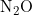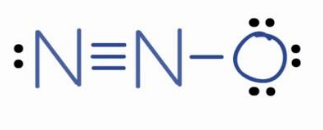×
Get Full Access to Introductory Chemistry - 5 Edition - Chapter 10 - Problem 71p
Get Full Access to Introductory Chemistry - 5 Edition - Chapter 10 - Problem 71p

×

# Determine the bond angles for each molecule in.(a) N2O (oxygen is terminal)(b) SO2(c)ISBN: 9780321910295 34

## Solution for problem 71P Chapter 10

Introductory Chemistry | 5th Edition

• Textbook Solutions
• 2901 Step-by-step solutions solved by professors and subject experts
• Get 24/7 help from StudySoup virtual teaching assistantsIntroductory Chemistry | 5th Edition

4 5 1 324 Reviews
17
5
Problem 71P

Determine the bond angles for each molecule in Problem 69.

Step-by-Step Solution:

Step 1 of 4

Here, we are going to determine the bond angles for the given molecules.

Bond angle is defined as the angle between the orbitals containing bonding electron pairs around the central atom in a molecule.

a)Number of valence electrons in N = 5

Number of valence electrons in O = 6

Total number of valence electrons in= (25) + 6 = 16

The 18 electrons must be distributed in such a way that all the atoms get 8 electron each. The lewis structure for the molecule is:From the above structure, it is clear that N2O has linear structure. Thus, the bond angle inis 180°.

Step 2 of 4

Step 3 of 4

##### ISBN: 9780321910295

Since the solution to 71P from 10 chapter was answered, more than 4392 students have viewed the full step-by-step answer. The full step-by-step solution to problem: 71P from chapter: 10 was answered by , our top Chemistry solution expert on 05/06/17, 06:45PM. The answer to “?Determine the bond angles for each molecule in 69.” is broken down into a number of easy to follow steps, and 9 words. This textbook survival guide was created for the textbook: Introductory Chemistry, edition: 5. This full solution covers the following key subjects: Angles, Bond, determine, molecule, oxygen. This expansive textbook survival guide covers 19 chapters, and 2046 solutions. Introductory Chemistry was written by and is associated to the ISBN: 9780321910295.

Unlock Textbook Solution